Maths-
General
Easy

Question

# Nikita invests  Rs6000 for two years at a certain rate of interest compounded annually. At the end of the 1st year, it amounts to Rs6720. Calculate (i). the rate of interest (ii) the amount at the end of 2nd yearHint:

## The correct answer is: 7526.4 Rupees

### Complete step by step solution:Let the rate of interest = R(i)  We know that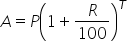…(i)Here, we have T = 1 year, P = 6000, A = 6720 and R = ?On substituting the known values in (i), we get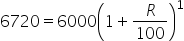On dividing both the sides by 6000, we have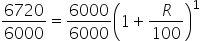(ii) We know that…(ii)Here, we have T = 2 years, P = 6000, R = 12Amount at the end of second year will be,On substituting the known values in (ii), we get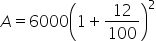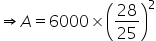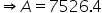RupeesSo the amount to be paid at the end of 2 years = 7526.4 Rupees.#### With Turito Foundation.#### Get an Expert Advice From Turito.